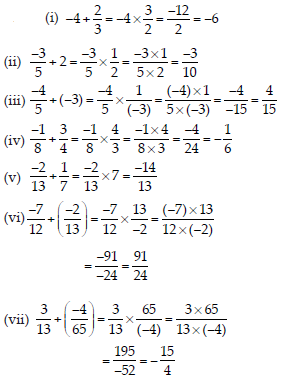# Rational Numbers (Maths) Class 7 - NCERT Questions

Q 1.

List five rational numbers between:
(i) –1 and 0
(ii) –2 and –1
(iii) -4/5 and -2/3
(iv) 1/2 and 2/3

SOLUTION: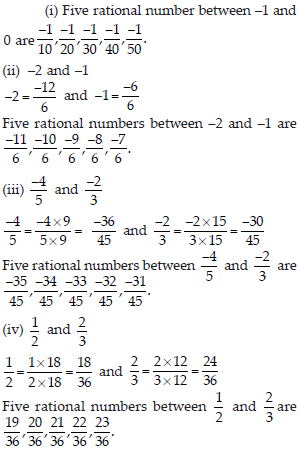Q 2.

Write four more rational numbers in each of the following patterns: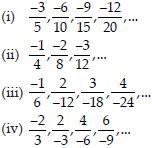SOLUTION: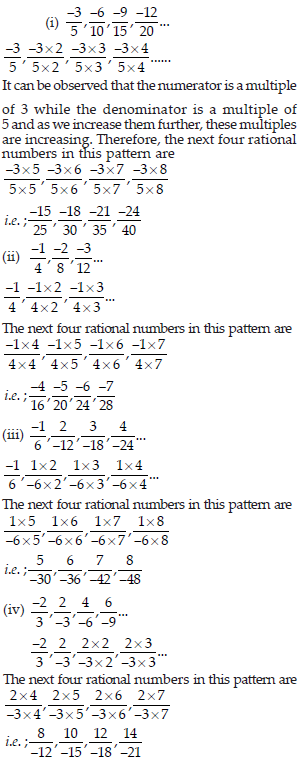Q 3.

Give four rational numbers equivalent to:
(i) -2/7
(ii) 5/-3
(iii) 4/9

SOLUTION: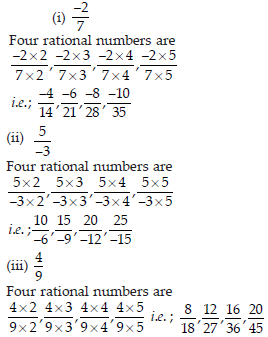Q 4.

Draw the number line and represent the following rational numbers on it:
(i) 3/4
(ii) -5/8
(iii) -7/4
(iv) 7/8

SOLUTION: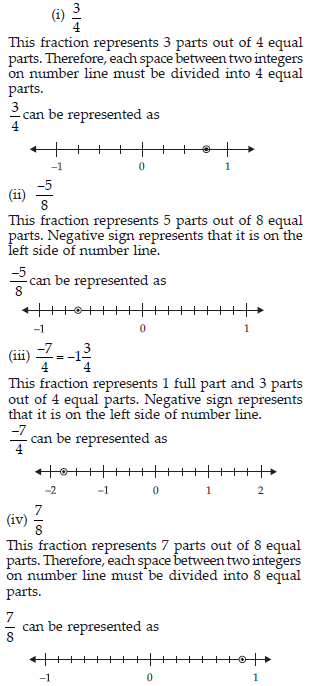Q 5.

The points P, Q, R, S, T, U, A and B on the number line are such that, TR = RS = SU and AP = PQ = QB. Name the rational numbers represented by P, Q, R and S.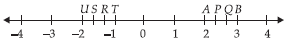SOLUTION: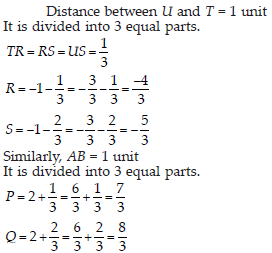Q 6.

Which of the following pairs represent the same rational number?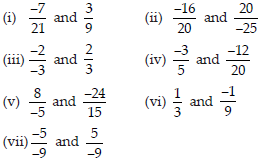SOLUTION: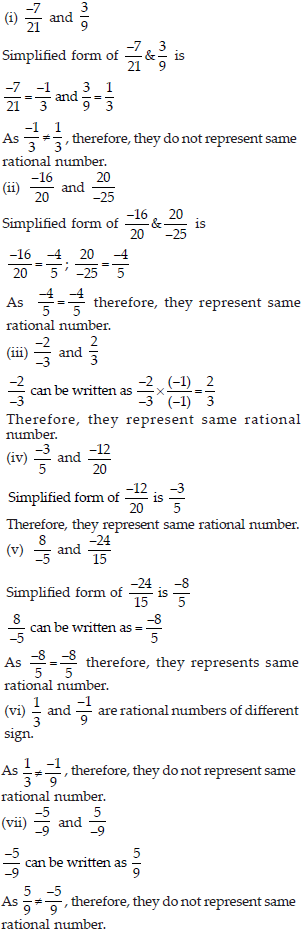Q 7.

Rewrite the following rational numbers in the simplest form: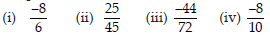SOLUTION: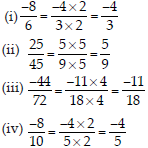Q 8.

Fill in the boxes with the correct symbol out of >, < and =.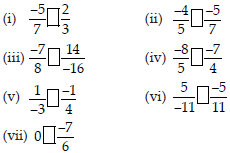SOLUTION: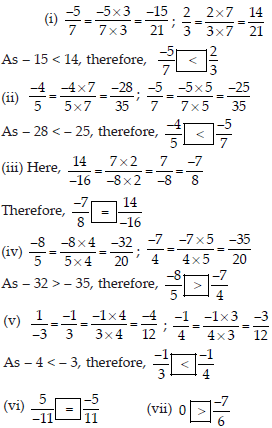Q 9.

Which is greater in each of the following: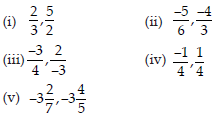SOLUTION: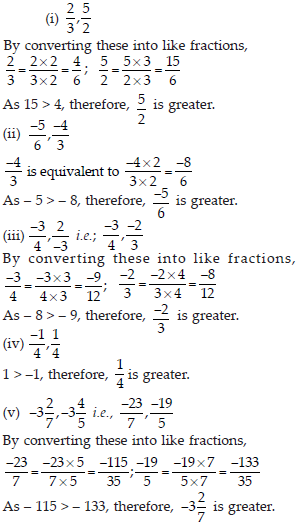Q 10.

Write the following rational numbers in ascending order: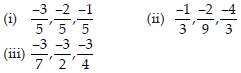SOLUTION: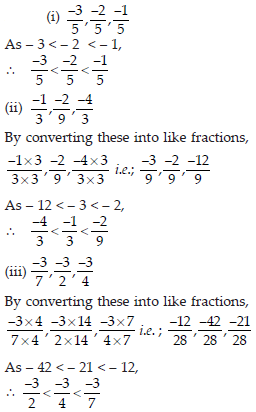Q 11.

Find the sum: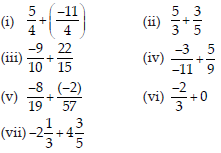SOLUTION: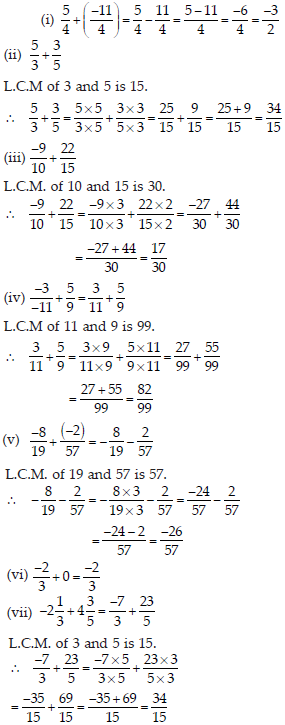Q 12.

Find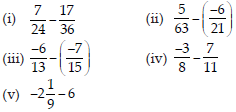SOLUTION: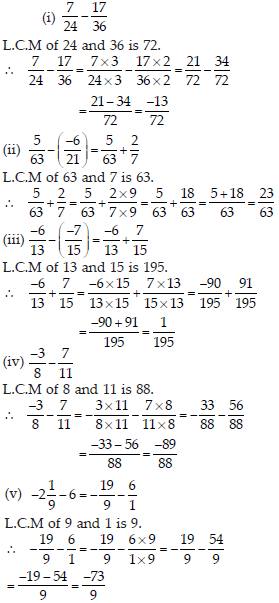Q 13.

Find the product: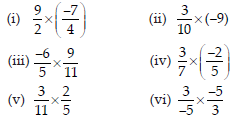SOLUTION: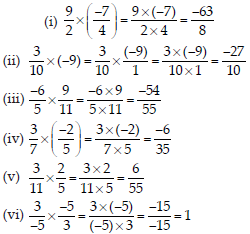Q 14.

Find the value of: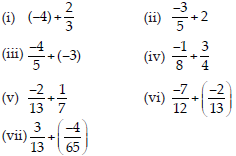SOLUTION: